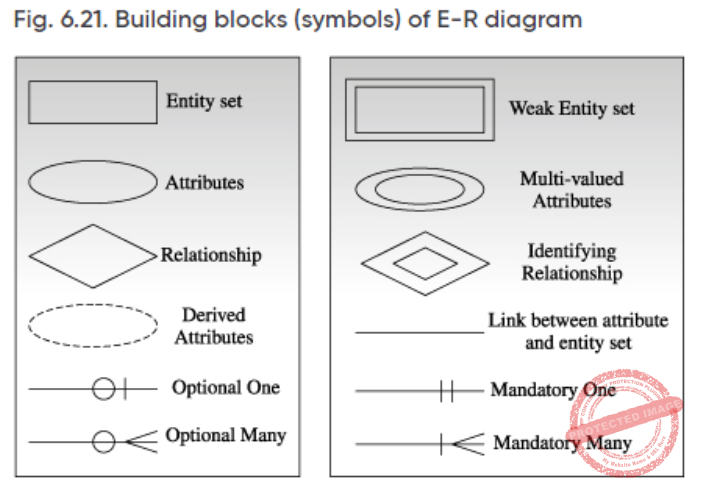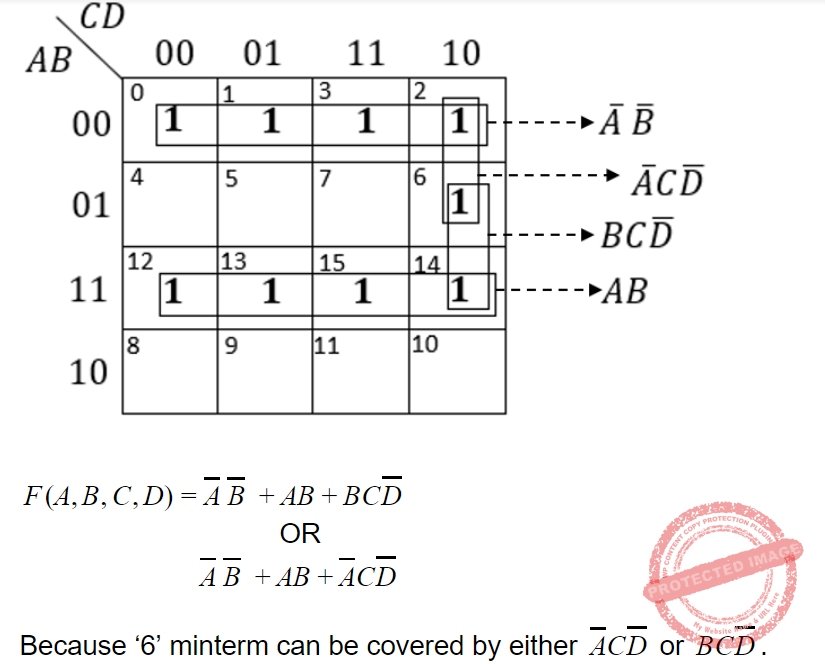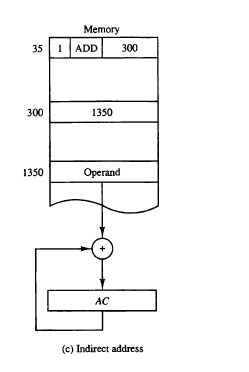## UGC NET JRF November 2020 Paper-2

 Question 1
A company has a choice of two language L1 and L2 to develop a software for their client. Number of LOC required to develop an application in L2is thrice the LOC in language L1. Also, software has to be maintained for the next 10 years. Various parameters for two languages are given below to decide which language should be preferred for development.
 PARAMETER L1 L2 Man-year needed for development LOC/1000 LOC/1000 Development cost Rs.70,000 Rs.90,000 Cost of Maintenance per year Rs.1,00,000 Rs.40,000
Total cost of the project includes the cost of development and maintenance. What is the LOC for L1for which cost of developing the software with both languages must be the same?
 A 2000 B 6000 C 3000 D 5000
Software-Engineering       LOC
Question 1 Explanation:
Let LOC of L1=X, so LOC of L2=3X
→ (X/1,000)*70,000 + 10*1,00,000 = (3X/1,000)*90,000 + 10*40,000
→70X+1000000=270X+400000
→200X=600000
→X=3000
 Question 2
The data node and name node in HADOOP are
 A Worker Node and Master Node respectively B Master Node and Worker Node respectively C Both Worker Nodes D Both Master Nodes
Question 2 Explanation:
Explanation: Hadoop Distributed File System(HDFS) has a master/slave architecture. An HDFS cluster consists:
1. NameNode, a master server that manages the file system namespace and regulates access to files by clients.
2. DataNodes, usually one per node in the cluster, which manage storage attached to the nodes that they run on. HDFS exposes a file system namespace and allows user data to be stored in files.
Internally, a file is split into one or more blocks and these blocks are stored in a set of DataNodes. The NameNode executes file system namespace operations like opening, closing, and renaming files and directories. It also determines the mapping of blocks to DataNodes. The DataNodes are responsible for serving read and write requests from the file system’s clients. The DataNodes also perform block creation, deletion, and replication upon instruction from the NameNode.Question 3
Suppose you have a Linux file system where the block size is 2K bytes, a disk address is 32 bits, and i-node contains the disk addresses of the first 12 direct blocks of file, a single indirect block, and a double indirect block. Approximately, what is the largest file that can be represented by an i-node?
 A 513 Kbytes B 513 MBytes C 537 Mbytes D 537 KBytes
Operating-Systems       File-system
Question 3 Explanation:Question 4
Match List I with List II.,Choose the correct answer from the options given below:
 A A-II, B-IV, C-III, D-I B A-IV, B-I, C-II, D-III C A-II, B-I, C-IV, D-III D A-III, B-IV, C-I, D-II
Database-Management-System       ER-Model
Question 4 Explanation:Question 5
Which of the following statements is true?
 A The union of two context free languages is context free. B The intersection of two context free languages is context free. C The complement of a context free language is context free. D If a language is context free, it can always be accepted by a deterministic pushdown automaton.
Theory-of-Computation       Context-Free-Language
Question 5 Explanation:Option 4 is incorrect because CFL includes both DCFL and NDCFL and NDCFL are accepted by only non-deterministic pushdown automata, they can’t be accepted by Deterministic PDA.
 Question 6
Consider the following languages: L1={aźZ| ź is an integer} L2={a | ź>0} L3={ωω| ω{a,b}*} Which of the languages is (are) regular?
 A L1 and L2 only B L1 and L3 only C L1 only D L2 only E None of the above
Theory-of-Computation       Regular-Language
Question 6 Explanation:
A language is Regular language only if it creates an AP series.
Here only statement 2 makes an AP series. Example: L be a lanusage where i=1 and Z>= 0 then L ={ epsilon, a, aa, aaa, aaaa,..................} which is an AP. And Finite automata for this will beQuestion 7
Arrange the following types of Cohesion from best to worst type. A) Logical Cohesion B) Sequential Cohesion C) Communication Cohesion D) Temporal Cohesion E) Procedural Cohesion Choose the correct answer from the options given below:
 A A➡ D➡ E➡ C➡ B B A ➡ E ➡D ➡ C ➡ B C B ➡ E ➡ C ➡ D ➡ A D B ➡ C ➡ E ➡ D ➡ A
Software-Engineering       Coupling-and-Cohesion
Question 7 Explanation:
➡ Cohesion Cohesion is a measure of the relative functional strength of a module.
➡ Types of cohesion :
➡ Coincidentally cohesive(LOW) a module that performs a set of tasks that relate to each other loosely, if at all. Such modules are termed coincidentally cohesive.
➡ Logically cohesive A module that performs tasks that are related logically (e.g., a module that produces all output regardless of type) is logically cohesive.
➡ Temporal cohesion When a module contains tasks that are related by the fact that all must be executed with the same span of time, the module exhibits temporal cohesion
➡ Procedural cohesion When processing elements of a module are related and must be executed in a specific order, procedural cohesion exists.
➡ Communicational cohesion When all processing elements concentrate on one area of a data structure, communicational cohesion is present.
➡ Sequential Cohesion If the function of the module forms part of sequence, where the output of one function is the input to another function.
➡ Functional Cohesion(HIGH): In this, different elements of the module cooperate with each other to achieve a single function.
 Question 8
Consider the statement below. A person who is radical (R) is electable (E) if he/she is conservative (C), but otherwise is not electable. Few probable logical assertions of the above sentence are given below.,Which of the above logical assertions are true?
Choose the correct answer from the options given below:
 A (B) only B (C) only C (A) and (C) only D (B) and (D) only
Artificial-intelligence       Propositional-Logic
Question 8 Explanation:
1. (R ∧E) ↔C
This is not equivalent. It says that all (and only) conservatives are radical and electable.
2. R →(E ↔C)
This one is equivalent. if a person is a radical then they are electable if and only if they are conservative.
3. R →((C →E) ∨¬E)
This one is vacuous. It’s equivalent to ¬R ∨ (¬C ∨ E ∨ ¬E), which is true in all interpretations.
4.R ⇒ (E ⇐⇒ C) ≡ R ⇒ ((E ⇒ C) ∧ (C ⇒ E))
≡ ¬R ∨ ((¬E ∨ C) ∧ (¬C ∨ E))
≡ (¬R ∨ ¬E ∨ C) ∧ (¬R ∨ ¬C ∨ E))
 Question 9
Consider the following argument with premiseA This is a valid argument. B Steps (C) and (E) are not correct inferences C Steps (D) and (F) are not correct inferences D Step (G) is not a correct inference
Artificial-intelligence       Propositional-Logic
 Question 10
Simplified expression/s for following Boolean function F(A,B,C,D)=Σ(0,1,2,3,6,12,13,14,15) is/are A) A'B'+AB+A'C'D' B) A'B'+AB+A'CD' C) A'B'+AB+BC'D' D) A'B'+AB+BCD' Choose the correct answer from the options given below:
 A (A) only B (B) only C (A) and (B) only D (B) and (D) only
Digital-Logic-Design       K-Map
Question 10 Explanation:Question 11
Given below are two statements: Statement I: In Caesar Cipher each letter of Plain text is replaced by another letter for encryption. Statement II: Diffie-Hellman algorithm is used for exchange of secret key. In the light of the above statements, choose the correct answer from the options given below
 A Both Statement I and Statement II are true B Both Statement I and Statement II are false C Statement I is correct but Statement II is false D Statement I is incorrect but Statement II is true
Computer-Networks       Network-Security
Question 11 Explanation:
In cryptography, a Caesar cipher, also known as Caesar's cipher, the shift cipher, Caesar's code or Caesar shift, is one of the simplest and most widely known encryption techniques. It is a type of substitution cipher in which each letter in the plaintext is replaced by a letter with some fixed number of positions down the alphabet. For example, with a left shift of 3, D would be replaced by A, E would become B, and so on. The method is named after Julius Caesar, who used it in his private correspondence.
Diffie–Hellman key exchange is a method of securely exchanging cryptographic keys over a public channel and was one of the first public-key protocols as conceived by Ralph Merkle and named after Whitfield Diffie and Martin Hellman
 Question 12
Consider a single-level page table system, with the page table stored in the memory. If the hit rate to TLB is 80%, and it takes 15 nanoseconds to search the TLB, and 150 nanoseconds to access the main memory, then what is the effective memory access time, in nanoseconds?
 A 185 B 195 C 205 D 175
Operating-Systems       Virtual Memory
Question 12 Explanation:
Effective memory access time =0.8(15+150)+0.2(15+150+150)
=132+63
=195 ns
 Question 13
A non-pipeline system takes 50ns to process a task. The same task can be processed in a six-segment pipeline with a clock cycle of 10ns. Determine approximately the speedup ratio of the pipeline for 500 tasks.
 A 6 B 4.95 C 5.7 D 5.5
Computer-Organization       Pipelining
Question 13 Explanation:
Time required to execute 500 tasks without pipelining =500*50 ns=25000 ns
Time required to execute 500 tasks with 6-stage pipelining =(1*6*10)+(499*1*10)
=60+4990
=5050Question 14
Given below are two statements: Statement I: 5 divides n5-n whenever n is a nonnegative integer. Statement II: 6 divides n3-n whenever n is a nonnegative integer. In the light of the above statements, choose the correct answer from the options given below
 A Both Statement I and Statement II are correct B Both Statement I and Statement II are incorrect C Statement I is correct but Statement II is incorrect D Statement I is incorrect but Statement II is correct
Engineering-Mathematics       Number-Systems
Question 14 Explanation:
Statement 1 is true. For example: Let n= 2 then n5-n will be 30 which is divisible by 5. Hence This statement is correct.
Statement 2 is also correct. For example: Let n= 2 then n3-n will be 6 which is divisible by 6. Hence This statement is correct.
 Question 15
Which of the following cloud concept/s is/are related to pooling and sharing of resources? A) Virtual Memory B) Service C) Virtualization Choose the correct answer from the options given below:
 A (C) only B (A) and (B) only C (A) only D (B) only
Operating-Systems       Distributed-Operating-System
Question 15 Explanation:
The availability of high-capacity networks, low-cost computers and storage devices as well as the widespread adoption of hardware virtualization, service-oriented architecture and autonomic and utility computing has led to growth in cloud computing.
Cloud computing is the on-demand availability of computer system resources, especially data storage (cloud storage) and computing power, without direct active management by the user. The term is generally used to describe data centers available to many users over the Internet
 Question 16
Using 'RSA' public key cryptosystem, if p=3, q=11 and d=7, find the value of e and encrypt the number '19'
 A 20,19 B 33,11 C 3,28 D 77,28
Computer-Networks       RSA-algorithm
Question 16 Explanation:
n= P*q
n= 3*11
n=33
ф(n) = (p-1)(q-1)
ф(n) = 2*10
ф(n) = 20
E * d=1 mod ф(n)
e*7=1 mod 20
Now find the value of “e” in such a way that if we multiply the value of “e” with 7 then by dividing it with 20 mod value should come as “1”.
So if e=3 then the above condition can be satisfied hence option C is the right answer. Encrypted value = (Message)e mod n = (19)3 mod 33 = 28
 Question 17
Consider the following regular expressions: a) r=a(b+a)* b) s=a(a+b)* c) t=aa*b Choose the correct answer from the options given below based on the relation between the languages generated by the regular expressions above:
 A L(r)⊆L(s)⊆L(t) B L(r)⊇L(s)⊇L(t) C L(r)⊇L(t)⊇L(s) D L(s)⊇L(t)⊇L(r)
Theory-of-Computation       Regular-Expression
Question 17 Explanation:
r={a,aa,ab,aaa,aab,aba,aaab,......}
s={aa,ab,aaa,aab,aba,aaab,......}
t={ab,aab,aaab,....}
If you notice then r⊇s⊇t
 Question 18
Firewall is a device that filters access to the protected network from the outside network. Firewall can filter the packets on the basis of (A) Source IP address (B) Destination IP Address (C) TCP Source Port (D) UDP Source Port (E) TCP Destination Port Choose the correct answer from the options given below:
 A (A), (B) and (C) only B (B) and (E) only C (C) and (D) only D (A), (B), (C), (D) and (E) only
Computer-Networks       Network-Security
Question 18 Explanation:
In computing, a firewall is a network security system that monitors and controls incoming and outgoing network traffic based on predetermined security rules. A firewall typically establishes a barrier between a trusted network and an untrusted network, such as the Internet.
Packets may be filtered by source and destination IP addresses, protocol, source and destination ports. The bulk of Internet communication in 20th and early 21st century used either Transmission Control Protocol (TCP) or User Datagram Protocol (UDP) in conjunction with well-known ports, enabling firewalls of that era to distinguish between specific types of traffic such as web browsing, remote printing, email transmission, file transfer.
 Question 19
Assuming that the system call fork () never fails, consider the following C programs P1 and P2 executed on a UNIX/ Linux system:Statement I: P1 displays “Happy” 8 times.
Statement II: P2 displays “Happy” 12 times.
In the light of the above statements, choose the correct answer from the options given below:
 A Both Statement I and Statement II are true B Both Statement I and Statement II are false C Statement I is correct but Statement II is false D Statement I is incorrect but Statement II is true
Operating-Systems       System-Calls
Question 19 Explanation:
As we know that “n” fork calls will lead to 2n-1child processes. Hence Total no. of process will be 2n-1+1(We added 1 for the parent process).
Now since in P1 all 3 fork calls are done before the printf statement so all 8( 23-1+1) processes will execute the Printf statement. Hence in P1 Printf statement will be executed for 8 times.
 Question 20
Consider a machine with a byte addressable main memory of 2616 bytes and block size of 8 bytes. Assume that a direct mapped cache consisting of 32 lines is used with this machine. How many bits will be there in Tag, line and word field of format of main memory addresses?
 A 8,5,3 B 8,6,2 C 7,5,4 D 7,6,3
Computer-Organization       Cache
Question 20 Explanation:Question 21
Let G be a simple undirected graph, TDbe a DFS tree on G, and TB be the BFS tree on G. Consider the following statements. Statement I: No edge of G is a cross with respect to TD Statement II: For every edge (u,v) of G, if u is at depth i and v is at depth j in TB then |i-j|=1 In the light of the above statements, choose the correct answer from the options given below
 A Both Statement I and Statement II are true B Both Statement I and Statement II are false C Statement I is correct but Statement II is false D Statement I is incorrect but Statement II is true
Data-Structures       BFS-and-DFS
Question 21 Explanation:
I. TRUE: Undirected graphs do not have cross edges in DFS. But can have cross edges in directed graphs.
II. FALSE: Just draw a triangle ABC. Source is A. Vertex B and C are at the same level at distance 1. There is an edge between B and C too. So here |i - j| = |1 - 1| = 0.
 Question 22
The process of removing details from a given state representative is called___.
 A Extraction B Mining C Selection D Abstraction
Database-Management-System       Data-ware-housing
Question 22 Explanation:
Abstraction: the process of removing physical, spatial, or temporal details or attributes in the study of objects or systems to focus attention on details of greater importance; it is similar in nature to the process of generalization.
Data extraction is the act or process of retrieving data out of (usually unstructured or poorly structured) data sources for further data processing or data storage (data migration).
Data mining is a process of discovering patterns in large data sets involving methods at the intersection of machine learning, statistics, and database systems.
 Question 23
What kind of clauses are available in conjunctive normal form?
 A Disjunction of literals B Disjunction of variables C Conjunction of literals D Conjunction of variables
Digital-Logic-Design       Logic-Gates
Question 23 Explanation:
In Boolean logic, a formula is in conjunctive normal form (CNF) or clausal normal form if it is a conjunction of one or more clauses, where a clause is a disjunction of literals; otherwise put, it is a product of sums or an AND of ORs.
 Question 24
Find the lexicographic ordering of the bit strings given below based on the ordering 0 < 1. A) 001 B) 010 C) 011 D) 0001 E) 0101 Choose the correct answer from the options given below:
 A 001<010<011<0001<0101 B 0001<001<010<0101<011 C 0001<0101<001<010<011 D 001<010<0001<0101<011
Algorithms       Sorting
Question 24 Explanation:
To find the lexicographic order of given strings will be:
0001<001<010<0101<011
Note: Lexicographical order is nothing but dictionary based order.
 Question 25
Given below are two statements: Statement I: hardwired control unit can be optimized to produce fast mode of operation. Statement II: Indirect addressing mode needs two memory references to fetch the operand. In the light of the above statements, choose the correct answers from the options given below
 A Both Statement I and Statement II are true B Both Statement I and Statement II are false C Statement I is correct but Statement II is false D Statement I is incorrect but Statement II is true
Question 25 Explanation:
Hardwired Unit:
It can be optimized to produce fast modes of operations.
It uses a large number of registers and because of that it is costly.
Instruction size in the Hardwired unit is fixed.
It has a small number of addressing modes.